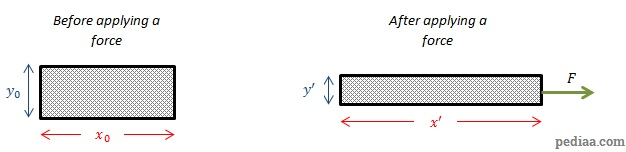# Difference Between Modulus of Elasticity and Modulus of Rigidity

## Main Difference – Modulus of Elasticity vs. Modulus of Rigidity

Modulus of elasticity and modulus of rigidity are two numbers that are used by materials engineers to describe how a material gets deformed. The main difference between modulus of elasticity and modulus of rigidity is that the modulus of elasticity describes how a material gets deformed when a force is applied at right angles to a surface of an object, causing the material to elongate or shorten while the modulus of rigidity describes how a material gets deformed when a force is applied parallel to a surface of an object, causing one of the surfaces to become shifted with respect to another surface of the same object.

## What is the Modulus of Elasticity

Modulus of elasticity (Young modulus) is a number that describes the ratio of stress to strain in an object that is being deformed by a force that is perpendicular to a surface of an object. The stress of a material is the deforming force per unit area. For instance, the figure below shows an object that becomes elongated as a result of a tensile force on it. In this case, the stress ($\sigma$) is given by:$\sigma=\frac{F}{A}$

Since the deforming force acts at right angles to the face of the object, the stress is often called normal stress.

The strain is the fractional change in length of the object. Suppose the object had a length$x_0$ before the deforming force acted on it, and if the object gets elongated by a length$\Delta x$ under the deforming force, then the strain ($\varepsilon$) is given by:$\varepsilon=\frac{\Delta x}{x_0}$

The modulus of elasticity ($E$) is then given by:$E=\frac{\sigma}{\varepsilon}$

## What is the Modulus of Rigidity

Modulus of rigidity (shear modulus) is a number that gives the shear stress acting on a material per unit area. Here, the deforming force acts parallel to a face of the object, causing one face to become displaced with respect to another face. This is depicted below:

So, shear stress ($\sigma$) is given as:$\sigma=\frac{F}{A}$

This equation has the same form as the equation for normal stress, the difference is in the way the force acts.

The shear strain ($\varepsilon$) is defined as the ratio of relative displacement between the surfaces to the separation between the surfaces. Here,$\varepsilon=\frac{\Delta x}{l}$

Once again the shear modulus ($G$) is the ratio between shear stress and shear strain:$G=\frac{\sigma}{\varepsilon}$

## Relationship between Modulus of Elasticity and Modulus of Rigidity

Modulus of elasticity ($E$) and the modulus of rigidity ($G$) are related by the following equation:$E=2G\left( 1+\nu\right)$

Here,$\nu$ represents a number called Poisson’s ratio given to the particular material. When the material is being stretched in one direction, it gets shortened in a perpendicular direction. In the direction that the material becomes elongated, the axial strain ($\varepsilon_a$) is defined as the fractional increase in the length. In the direction that the material shortens, the transverse strain ($\varepsilon_t$) gives the fractional reduction in length. The diagram below illustrates these changes in shape:Defining Poisson’s ratio

In this diagram, the axial strain is:$\varepsilon_a=\frac{x'-x_0}{x_0}$

The transverse strain is:$\varepsilon_t=\frac{y'-y_0}{y_0}$

Note that since the object shortens in the directiopn perpendicular to the force,$\varepsilon_t<0$. Poisson’s ratio ($\nu$) is defined as:$\nu=-\frac{\varepsilon_t}{\varepsilon_a}$

The minus sign has been introduced to ensure that$\nu$ takes a positive value.

## Difference Between Modulus of Elasticity and Modulus of Rigidity

### Direction of Force

Modulus of elasticity is used to calculate the deformation of an object when a deforming force acts at right angles to a surface of the object.

Modulus of rigidity is used to calculate deformations when a deforming force acts parallel to the surface of an object.

### Change in Shape

Where modulus of elasticity is calculated, the object under the deforming force either gets lengthened or shortened.

Where modulus of rigidity is calculated, one of the surfaces of the object becomes displaced with respect to another surface.

### Relative Size

For most materials, the modulus of elasticity is larger than the modulus of rigidity. The exceptions to this rule are the so-called “auxetic” materials which have negative Poisson’s ratios, but these materials are less common.